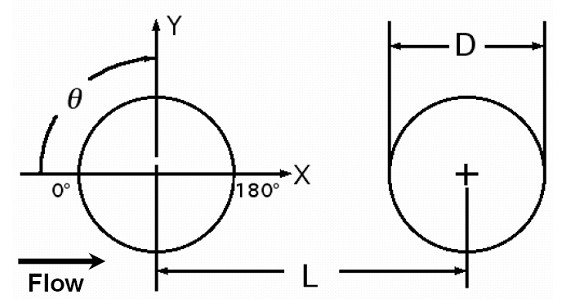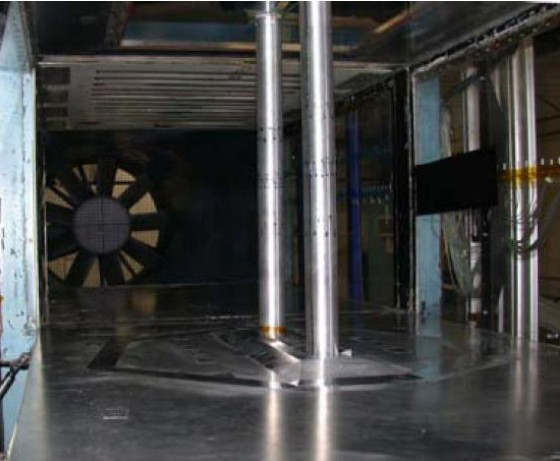# Test Case Study

## Brief Description of the Study Test Case

A detailed description of the chosen test case (TC with L = 3.7D) is available here. So in the following we present only its brief overview.

A schematic of the airflow past the TC configuration is shown in Figure 1. The model is comprised of two cylinders of equal diameter aligned with the streamwise flow direction. The polar angle, ${\theta }$, is measured from the upstream stagnation point and is positive in the clockwise direction.Figure 1: Schematic of TC configuration 

Geometric and regime parameters defining the test case are summarized in Table 1.

Table 1: Flow parameters
Parameter Notation Value
Reynolds number Re=$U_{0}D/\nu$1.66×105
Mach number M 0.128
Separation distance $L/D$3.7
TC aspect ratio $L_{z}/D$12.4
Cylinder diameter $D$0.05715 m
Free stream velocity $U_{0}$44 m/s
Free stream turbulence intensity $K$0.1%

The principal measured quantities by which the success or failure of CFD calculations are to be judged are as follows:

• Mean Flow
• Distributions of time-averaged pressure coefficient, $C_{p}=\langle (p-p_{0})\rangle /(1/2\rho _{0}U_{0}^{2})$, over the surface of both cylinders;
• Distribution of time-averaged mean streamwise velocity ${\left.\langle u\rangle /U_{0}\right.}$along a line connecting the centres of the cylinders;
• Distributions of the root-mean-square (rms) of the pressure coefficient over the surface of both cylinders;
• Power spectral density of the pressure coefficient (dB/Hz versus Hz) on the upstream cylinder at $\theta$= 135°;
• Power spectral density of the pressure coefficient (dB/Hz versus Hz) on the downstream cylinder at $\theta$= 45°;
• Turbulence kinetic energy
• x – y cut of the field of time-averaged two-dimensional turbulent kinetic energy ${\text{TKE}}={\frac {1}{2}}\left(\langle u'u'\rangle +\langle v'v'\rangle \right)/U_{0}^{2}$;
• 2D TKE distribution along y = 0;
• 2D TKE distribution along x = 1.5 D (in the gap between the cylinders);
• 2D TKE distribution along x = 4.45 D (0.75 D downstream of the centre of the rear cylinder).

All these and some other data are available here in both Windows and Unix compressed formats:

Windows Unix
data.zip data.tgz

These data are gratefully made available by the BANC-I Workshop.

## Test Case Experiments

A detailed description of the experimental facility and measurement techniques is given in the original publications [2-4]. So here we present only concise information about these aspects of the test case.Figure 2: TC configuration in the BART facility 

Experiments have been conducted in the Basic Aerodynamic Research Tunnel (BART) at NASA Langley Research Center (see Figure 2). This is a subsonic, atmospheric wind-tunnel for investigation of the fundamental characteristics of complex flow-fields. The tunnel has a closed test section with a height of 0.711 m, a width of 1.016 m, and a length of 3.048 m. The span size of the cylinders was equal to the entire BART tunnel height, thus resulting in the aspect ratio Lz / D = 12.4. The free stream velocity was set to 44 m/s giving a Reynolds number based on cylinder diameter equal to 1.66 × 105 and Mach number equal to 0.128 (flow temperature T = 292 K).

The free stream turbulence level was less than 0.10%. In the first series of the experiments [2, 3], in order to ensure a turbulent separation from the upstream cylinder at the considered Reynolds number, the boundary layers on this cylinder were tripped between azimuthal locations of 50 and 60 degrees from the leading stagnation point using a transition strip. For the downstream cylinder, it was assumed that trip-like effect of turbulent wake impingement from the upstream cylinder would automatically ensure turbulent separation. However, later on  it was found that the effect of tripping of the downstream cylinder at L/D = 3.7 is also rather tangible (resulted in reduced peaks in mean Cp distribution along the rear cylinder, accompanied by an earlier separation from the cylinder surface, a reduced pressure recovery, lower levels of mean TKE in the wake and reduced levels of peak surface pressure fluctuations). For this reason, exactly these (with tripping of both cylinders) experimental data  were used for the comparison with fully turbulent CFD.

In the course of experiments, steady and unsteady pressure measurements were carried out along with 2-D Particle Image Velocimetry (PIV) and hot-wire anemometry used for documenting the flow interaction around the two cylinders (mean streamlines and instantaneous vorticity fields, shedding frequencies and spectra).

Information on the data accuracy available in the original publications [2-4] is summarized in Table 2. Most absolute values are given based on nominal tunnel conditions or on an average data value. Percentage values are quoted for parameters where the uncertainty equations were posed in terms of the uncertainty relative to the nominal value of the parameter.

Table 2: Estimated Experimental Uncertainties
Quantity Uncertainty
Drag Coefficient 0.0005
PIV: Umean, Vmean 0.03 (normalized)
PIV: Spanwise velocity 1.8 (normalized)
PIV: TKE 4%
Power Spectral Density (PSD) 10 – 20%
Cp' rms 5 – 11%
Diameter, D; Sensor spacing Δz 0.005 inch

## CFD Methods

The key physical features of the UFR (see Description) present significant difficulties for all the existing approaches to turbulence representation, whether from the standpoint of solution fidelity (for the conventional (U)RANS models) or in terms of computational expense for full LES (especially if the turbulent boundary layers are to be resolved). For this reason, most of the computational studies of multi-body flows, in general, and the TC configuration, in particular, are currently relying upon hybrid RANS-LES approaches. This is true also with regard to simulations carried out in the course of the BANC-I and II Workshops and in the framework of the ATAAC project, where different hybrid RANS-LES models of the DES type were used (see Table 3) .

Table 3: Summary of simulations
Partner Turbulence Modelling approach Compressible/Incompressible Lz Grid Side Walls
Beijing Tsinghua University BTU SST DDES Compressible 3D Mandatory Slip
German Aerospace Center, Göttingen DLR SA DDES Compressible 3D Mandatory Slip
New Technologies and Services, St.-Petersburg, Russia NTS SA DDES

SA IDDES

Incompressible and Compressible 3D, 16D Mandatory Slip
Technische Universität Berlin TUB SA DDES

SA IDDES

Incompressible 3D Mandatory Slip
SST - k–ω Shear Stress Transport model ; SA - Spalart-Allmaras model ; SA and SST DDES - Delayed DES based on the SA and SST models ; SA IDDES - Improved DDES based on the SA model .

As mentioned in the experiments section above, in the experiments the boundary layers on both cylinders were tripped ahead of their separation, thus justifying the "fully turbulent" simulations.

All the partners used their own flow solvers.

Particularly, BTU employed in-house compressible Navier-Stokes code with weighted, central-upwind, approximation of the inviscid fluxes based on a modification of the high-order symmetric total variation diminishing scheme. The method combines 6th order central and 5th order WENO schemes. For the time integration, an implicit LU-SGS algorithm is applied with Newton-like sub-iterations.

DLR used their unstructured TAU code with a finite volume compressible Navier-Stokes solver. The solver employs a standard central scheme with matrix dissipation with dual time stepping strategy of Jameson. The 3W Multi-Grid cycle was applied for the momentum and energy equations, whilst the SA transport equation was solved on the finest grid only. Time integration was performed with the use of explicit 3-level Runge-Kutta scheme. The method is of the 2nd order in both space and time.

NTS used in-house NTS finite-volume code. It is a structured code accepting multi-block overset grids of Chimera type. The incompressible branch of the code employs Rogers and Kwak's scheme  and for compressible flows Roe scheme is applied. The spatial approximation of the inviscid fluxes within these methods is performed differently in different grid blocks (see Figure 3 below). In particular, in the outer block, the 3rd-order upwind-biased scheme is used, whereas in the other blocks, a weighted 5th-order upwind-biased / 4th-order central scheme with automatic (solution-dependent) blending function  is employed. For the time integration, implicit 2nd-order backward Euler scheme with sub-iterations was applied.

Finally, TUB applied their in-hose multi-block structured code ELAN in the framework of the incompressible flow assumption. The pressure velocity coupling is based on the SIMPLE algorithm. For the convective terms a hybrid approach  with blending of 2nd-order central and upwind-biased TVD schemes was used. The time integration was similar to that of NTS.

The viscous terms of the governing equations in all the codes are approximated with the 2nd order centered scheme.

All the simulations were carried out on the same, "mandatory", grid which X-Y cut is shown in Figure 3). This is a multi-block structured grid designed according to guidelines for DES-like simulations . The grid has 5 main blocks: 1 block in the outer or Euler Region (ER), 3 blocks in the Focus Region (FR), which includes the gap between the cylinders and the near wake of the downstream cylinder, and 1 block in the Departure Region (DR). The distance between the nodes on the forward half of the upstream cylinder is close to 0.02D and on its backward part it is 0.01D with smooth transition between the two. As a result, the total number of nodes on the surface of the upstream cylinder is 245. On the downstream cylinder there are 380 uniformly distributed nodes (the distance between the nodes is 0.008D). In the law of the wall units, the r-step closest to cylinders walls is less than 1.0. In the major part of the FR the cells are nearly isotropic with a size of about 0.02D. In the ER and DR the grid steps increase gradually (linearly with r). The total size of the grid in the XY plane is 82,000 cells.

According to recommendations of the organizers of the BANC-I Workshop, a mandatory spanwise size of the computational domain, Lz, in all the simulations was set equal to 3D. The grid-step in span-direction, Δz, was 0.02D, resulting in the nearly cubic cells in the focus region and a total number of cells about 11 million.

The boundary conditions used in the simulations were as follows.

No-slip conditions were imposed on the cylinders walls and periodic conditions were used in the z-direction, whereas the lateral boundaries were treated as frictionless walls (free-slip condition) in order to account for the blockage effect of the sidewalls of the experimental test-section at ±8.89D.

Inflow and outflow boundary conditions were different in the incompressible and compressible simulations. Particularly, in the compressible simulations of BTU and DLR characteristic boundary conditions were imposed on both inflow and outflow boundaries with no sponge layers, whereas NTS applied boundary conditions of characteristic type only at the inflow boundary, whereas at the outflow boundary a constant static pressure was specified, and the remaining flow parameters were extrapolated to the boundary from the interior of the domain. In order to avoid reflections of the waves from the outflow boundary, a sponge layer was used allocated to an additional Cartesian grid block of length 15D.

In the incompressible simulations of NTS a uniform velocity and constant pressure were specified at the inflow and outflow of the domain respectively.

1. Some participants of the BANC Workshops have used pure LES rather than DES-like approaches but these computations had the most difficulty simulating the high Reynolds aspects of the flow .

Contributed by: A. Garbaruk, M. Shur and M. Strelets — New Technologies and Services LLC (NTS) and St.-Petersburg State Polytechnic University